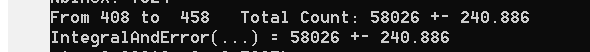# Comparison between DoIntegral and IntegralAndError Methods

Dear all,

Is there any documented example in ROOT website rather than a definition to see the difference in use?
I found one line for IntegralAndError method as below:
Double_t error;
Double_t integral = Hist->IntegralAndError(10, 70, error, “”); // “” … or … “width”
std::cout << "Hist. integral = " << integral << " ± " << error << std::endl;

//////////////////////////////// These are the definitions of the methods in short . Link is here.
IntegralAndError(int binx1, int binx2, double &error, Option *option="");
DoIntegral( int binx1, int binx2, double &err, Option *opt);
///////////////////////////////////
I almost don’t see any difference without looking at the actual line of long codes in the descriptions.
Again, what is the usage of this option pointer if we avoid (don’t understand) the lines of codes… I mean any example anywhere? Appreciated in advance.

Is this correct: all we need to do is define a variable in advance before calling the method because it knows what to do to retrieve the error.

Cheers.

TH1::DoIntegral is a protected member function (i.e., nothing for you).

Ok. Stick with the IntegralAndError method ,then. What about the option part!

Same as TH1::Integral

Is it just the binwidth that wee need to specify?
For instance, h1->Integral( 5, 15,???);

One another related question with integral method:
I wrote just a small part of the code to discuss to main idea.
////////////////////////////////////////////////////// 1ST PART //////////////////////////////////////////
int LL= 5, UL= 20;
for (int i = LL; i < UL+1 ; i++ ){

//cout<< i << " " << h1->GetBinContent(i) << endl;
TotalCounts += h1->GetBinContent(i);
TotalError += std::pow(h1->GetBinError(i), 2.);
}
TotalErrorUpdated= sqrt(TotalError);

cout <<“From” <<LL << " to " << UL <<" Total Count: " << TotalCounts <<" ± " << TotalErrorUpdated << endl;
//////////////////////////////////////////////////////// 2ND PART ////////////////////////////////
double myerror;
double myintegral = h1->IntegralAndError(LL, UL, myerror, “”); // “” … or … “width”
cout << "IntegralAndError(…) = " << myintegral << " ± " << myerror << endl;

///////////////////////////////////////////////////////////////////////// RESULTS ///////////////////////NOW, THE ERRORS MATCH.
DONE.

Cheers.

Options are character strings, i.e., `""` or `"width"` (uses histogram’s bins’ widths).

BTW. `TotalError += std::pow(h1->GetBinError(i), 2.);`

1 Like

The definition of the GetBinError is sigma value of the count in that bin, right?
For instance, N1 and N2 are count numbers.
sigma_total_square = var(N1+N2)=var(N1)+var(N2)
sigma1_square = var(N1)
sigma2_square= var(N2)
sigma_total = sqrt ( var(N1)+var(N2) ) = sqrt (sigma1_square + sigma2_square )
Thus, sigma_total should be equal to sqrt ( [h1->GetBinError(bin1)*h1->GetBinError(bin1)]+ [h1->GetBinError(bin1)*h1->GetBinError(bin1)] )

we can summarize as TotalError += std::pow(h1->GetBinError(i), 2.);
TotalErrorUpdated= sqrt (TotalError);

I think you forgot sqrt() , right?

BTW.
How should I use this string part so called option ?
For instance, h1->Integral( 5, 15,???);

This topic was automatically closed 14 days after the last reply. New replies are no longer allowed.